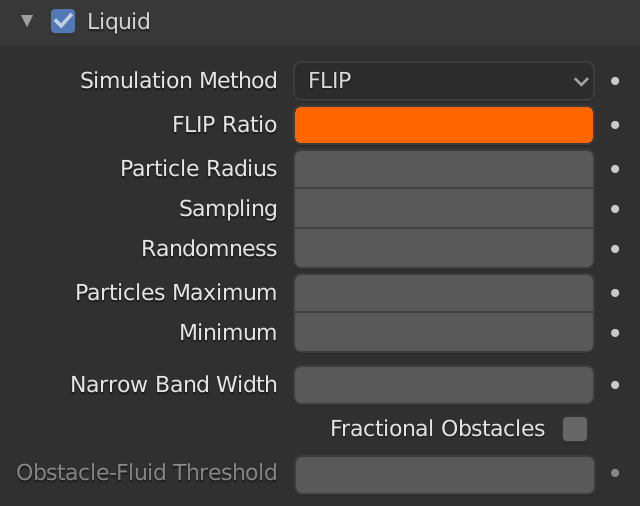# FLIP Ratio

– PIC (Particle in Cell) and FLIP (Fluid Implicit Particle) are two different methods blender uses to calculate the motions of the particles (more precisely the velocities).
PIC is less accurate, but stable. More PIC produces more viscous fluids as a consequence of numerical dissipation (due to a double interpolation).
FLIP better maintains individual particle velocities, is very accurate but can become chaotic and instable. More FLIP produces less viscous fluids and more noise on the surface.
PIC/FLIP is a hybrid method which mixes (linear combination) PIC and FLIP.
– Blender default value for Flip Ratio is 0.97 which gives good stability and accuracy.

– Flip Ratio=1.0 :a completely FLIP simulation (lot of splashes, more unstable)
– Flip Ratio=0.0 :a completely PIC simulation (less splashes, more stable)

– As always, remember: Blender is not “real simulations” oriented, we will never obtain a “real” simulation as everything is based on approximate physics that lead to approximate solutions, so choose the parameters that give you the simulation you like more!

– In this example see how 8 different values of Flip Ratio affect a simulation. Note how Flip Ratio=0 does not produce splashes while Flip Ratio=1 too many: